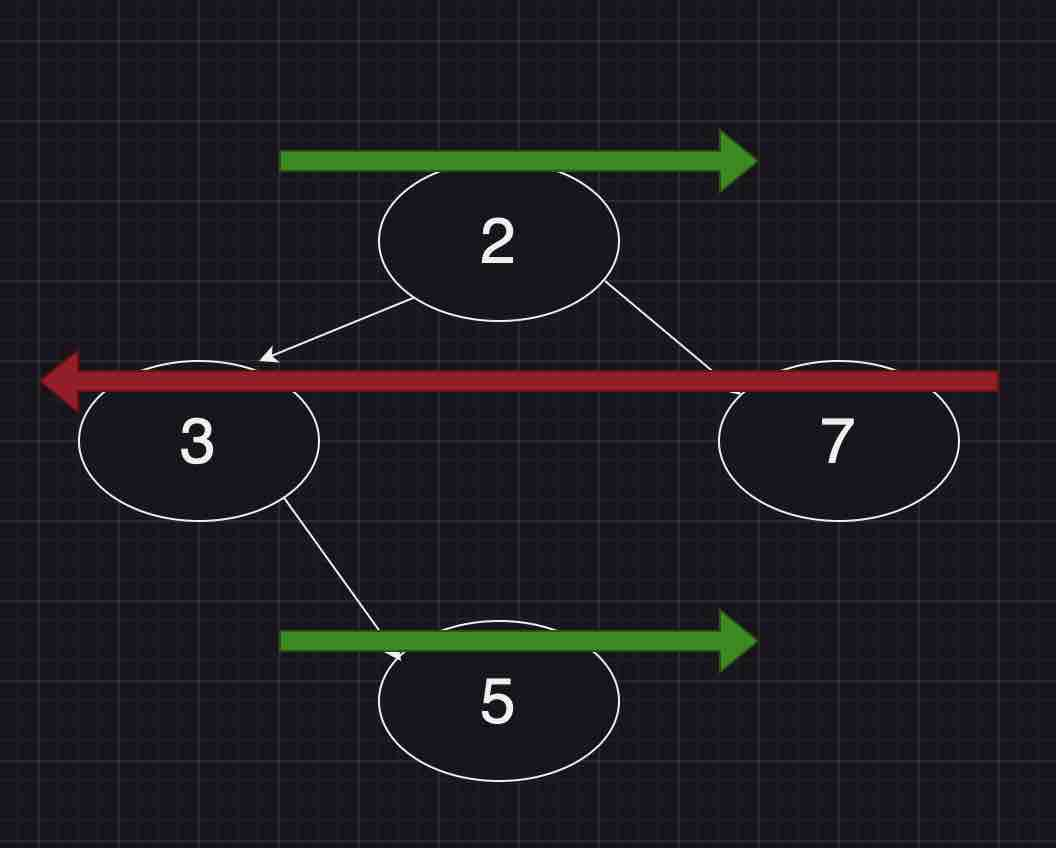Paul Coroneos
Published on

# Leetcode 103. Binary Tree Zigzag Level Order Traversal (JavaScript)

Today we will be reviewing Leetcode 103 - Binary Tree Zigzag Level Order Traversal which provides a good use case for breadth first traversal (BFS) of a tree.

## Problem Statement and analysis

Given the root of a binary tree, return the zigzag level order traversal of its nodes' values. (i.e., from left to right, then right to left for the next level and alternate between).

A bit confused? Let's consider a simple tree with some values and arrows superimposed:The arrows will represent the "zigzag" in which we form the answer. In other words, we want to write each level of the tree to a separate answer array in the opposite direction of the previous level the tree. Now it's important to note here that we still will traverse the tree with standard BFS methodology (using 2 queues implemented with JavaScript arrays).

So how can we represent which direction we should write the node values per level? The simplest solution I could come up with was simply using a boolean value `ltr` (left to right) which we will toggle after each level. For each level, we will collect the corresponding nodes into a subArray where once we have finished iterating through the entire level will push the entire result to the `ans` array.

## Solution and Explanation

``````/**
* Definition for a binary tree node.
* function TreeNode(val, left, right) {
*     this.val = (val===undefined ? 0 : val)
*     this.left = (left===undefined ? null : left)
*     this.right = (right===undefined ? null : right)
* }
*/
/**
* @param {TreeNode} root
* @return {number[][]}
*/
var zigzagLevelOrder = function(root) {
if(!root) return [];

let queue = [root]
const ans = [];
let ltr = true;

while(queue.length) {
const secondQueue = [];
const length = queue.length;
const subAns = [];
for(let i = 0 ; i < length; i++) {
const node = queue[i]
subAns.push(node.val)
if(node?.left) {
secondQueue.push(node.left)
}
if(node?.right) {
secondQueue.push(node.right);
}
}
ans.push(ltr ? subAns : subAns.reverse());
queue = secondQueue;
ltr = !ltr;
}

return ans;

};
``````

The first thing we want to do if first check to see if the tree even exists. If the root is null then there's no point in continuing and we can simply return `[]` as the answer. Otherwise, we start by initializing the queue with the root node. We set the `ltr` variable to `true` to indicate we want to write the first level's nodes in left to right order. We then will create a while loop that will run until the queue is empty. We also capture the length of the number of nodes for the level we are about to do work on to ensure we have pushed all child nodes of this level into the queue for work in the next while loop iteration. This is guaranteed by calculating the length of the number of nodes for the given level to ensure we do not "overwork" the current level and start working on nodes in subsequent levels.

Inside each while loop iteration, we start by creating a second queue which we initialize to an empty array. We then capture the current queue length (all nodes in the current level). Finally, we create a `subAns` array which will store intermediate information we will push to our overall answer (the values of the nodes for a given level in either left to right or right to left).

Now we perform pretty vanilla BFS. The action we perform on each node is to immediately push its `val` into the `subAns`array. We will do more work on this `subAns`array once we have iterated fully through our for loop. Then we check to see if there is a child node to the left of the current node. If there is we push that to the secondQueue (which will represent the next queue we will work on in the subsequent while loop iteration). If there is a right node present we push that as well. The reason we must check is that there is no guarantee a node can have a right child or even a left child. We repeat this until the queue is exhausted (we have iterated through all nodes in the level).

Now we need to update our answer array. We need to push the `subAns` we generated iterating through the for loop. However, first we need to check to see the state of the `ltr` variable. If it's true then we push the array as is. If it's false this means we want to print values from right to left in which case we perform a simple `Array.reverse()` on the `subAns` array before pushing it to the answer array. Finally, we update the queue to be the `secondQueue` we generated in the for loop. We also toggle the `ltr` variable to ensure we print the next level in the opposite direction of the previous level.

The above is repeated until we have visited each node in the tree. Once the while loop exists we will return the answer which contains the node values from the tree printed in "zigzag" order.

## Time and Space complexity

The time complexity of this solution is `O(n)` where `n` is the number of nodes in the tree. This is because we must visit each node in the tree to generate the answer. Space complexity is similarly `O(n)` because we must store each node in the tree in the queue to perform BFS.

Thank you for reading my explanation and best of luck in your studies!